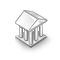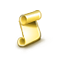Interesting script?
So post a link to it -
let others appraise it
You liked the script? Try it in the MetaTrader 5 terminal# ZH_Functions_Tools_1 - library for MetaTrader 4

|
Views:
5265
Rating:
(5)
Published:
2008.06.13 06:47
Updated:
2016.04.26 11:02

1.A function for automated distributing of color spectrum.
AutoColor (inversion of color distribution true/false = red-violet/violet-red,
true/false = filling an array/returning a fixed value (0 - 1535),
if ArraySingle true/false = amount of spectral lines/value (1 - 1535) for calculating a spectral line,
a one-dimension arrays to be filled for ArraySingle = true);
int AutoColor (bool InversColor, bool ArraySingle, int AmountSpectralLine, int Set_Color[]);
2. The function returns string name of a timeframe (TF) by TF number.
NumberInString (TF number from 0 to 8);
string NumberInString (int NumberTF);
3. A function to calculate the register length of a number before its decimal point.
Exponent (a number for calculations, a limitation on register length calculations);
The register length of a number in the format of 1.234х10^p is its exponent (p) with the base number of ten.
int Exponent (double Value, int Limiter);
4. Visualization of array data with double-precise data in comments.
DoubleArrayInComment (array name, the amount of decimal places to be visualized, 1-dimensional array to be visualized, 2-dimensional array to be visualized);
string DoubleArrayInComment (string NameArray, int digits, double Array1[], double Array2[][]);
5. Visualization of array data with integer data in comments.
IntArrayInComment (array name, 1-dimensional array to be visualized, 2-dimensional array to be visualized);
string IntArrayInComment (string NameArray, int Array1[], int Array2[][]);

Translated from Russian by MetaQuotes Ltd.
Original code: https://www.mql5.com/ru/code/7971i4 GoldenLionTrend v3

The indicator shows the trend. A code example for candlesticks colored in four different colors according to the trend strength.OutQuotingsKloss_MacdPatternTraderv01. +300 points monthly